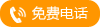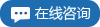•YD系列隔膜式液 `` ``
•无线压力变送器 `` ``
•高防护型温度变 `` ``
•电动真空源 `` ``
•雷达式水位计 `` ``
•双法兰液位变送 `` ``
•YD系列单晶硅智 `` ``
•台式液体压力源 `` ``
•电容式液位计 `` ``
•YTZ型电阻远传压 `` ``
•双法兰液位变送 `` ``
•YD系列单晶硅智 `` ``
•隔膜式双法兰液 `` ``
•YD系列单晶硅智 `` ``
•电容式液位变送 `` ``
•高防护型温度变 `` ``
•一体化温度变送 `` ``
•无线压力变送器 `` ``
•XY2009B电动真 `` ``
•浮球式压力计是 `` ``